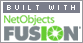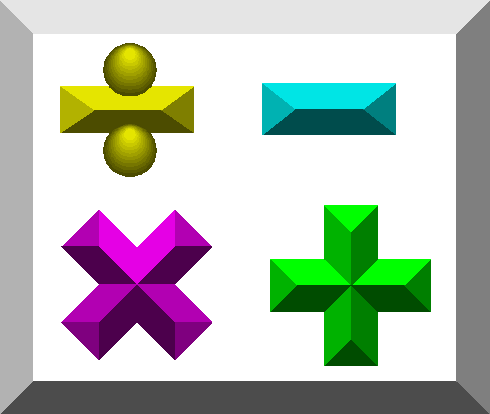Coming to Statistica
in 2022/2023

Zoom tutorials
using Explaineverything app
We a record sessions for you to listen to later

CALL STATISTICA NOW

.

E-mail     anne@statistica.com.au

# Place         Willetton, Western AustraliaPlease be aware the notes are my personal notes and solutions and are here to aid your learning Questions are from WashingtonHere are examples from M136 tutorials Tutorial Topic One            Revision, Indices, solving square root problems ie Ö126,                                            rationalising ‘opposite sign one’ Tutorial Topic Two            Algebra, powers (m2 n-2 /z n-4 m7 )4, Equations of Straight lines,                                            using two points, and point/intercept and gradient,                                            perpendicular and parallel lines    Tutorial Topic Six             Continuity of a function and piece-wise functions                                            the ones where the equation changes for different values of x Tutorial Topic Seven         Differentiation from First Principles,                                            Basic differentiation in Product/Quotient                                           Rules Displacement, Velocity and Acceleration Tutorial Topic Nine           Implicit Differentiation differentiating with respect to another letter                                             when you have two or  more letters i.e. dy/dt  du/dx Tutorial Topic Ten            Related rates, differentiating with respect to time Tutorial Topic Ten plus    Differentials dy/dx = dy/dx  and Linear Approximations f(x + dx ) = f ' (x) dx  + f(x) Tutorial Topic Eleven           Differentiation Max, Min, Inc, Dec, Concavity etc      Tutorial Topic Fourteen   Vectors  resultant vectors, adding vectors, angles between vectors Tutorial Topic Fifteen       Ships Colliding or not   Tutorial Topic Seventeen Matrices adding/subtracting, multiplication, Inverse of 2 x 2, Row elimination,                                           solving equations x -3y+ z =9 2x -3y+ 4z =19 2x -3z=2[Home] [Parents] [Contact us] [Services] [Mathematics] [Statistics] [School]